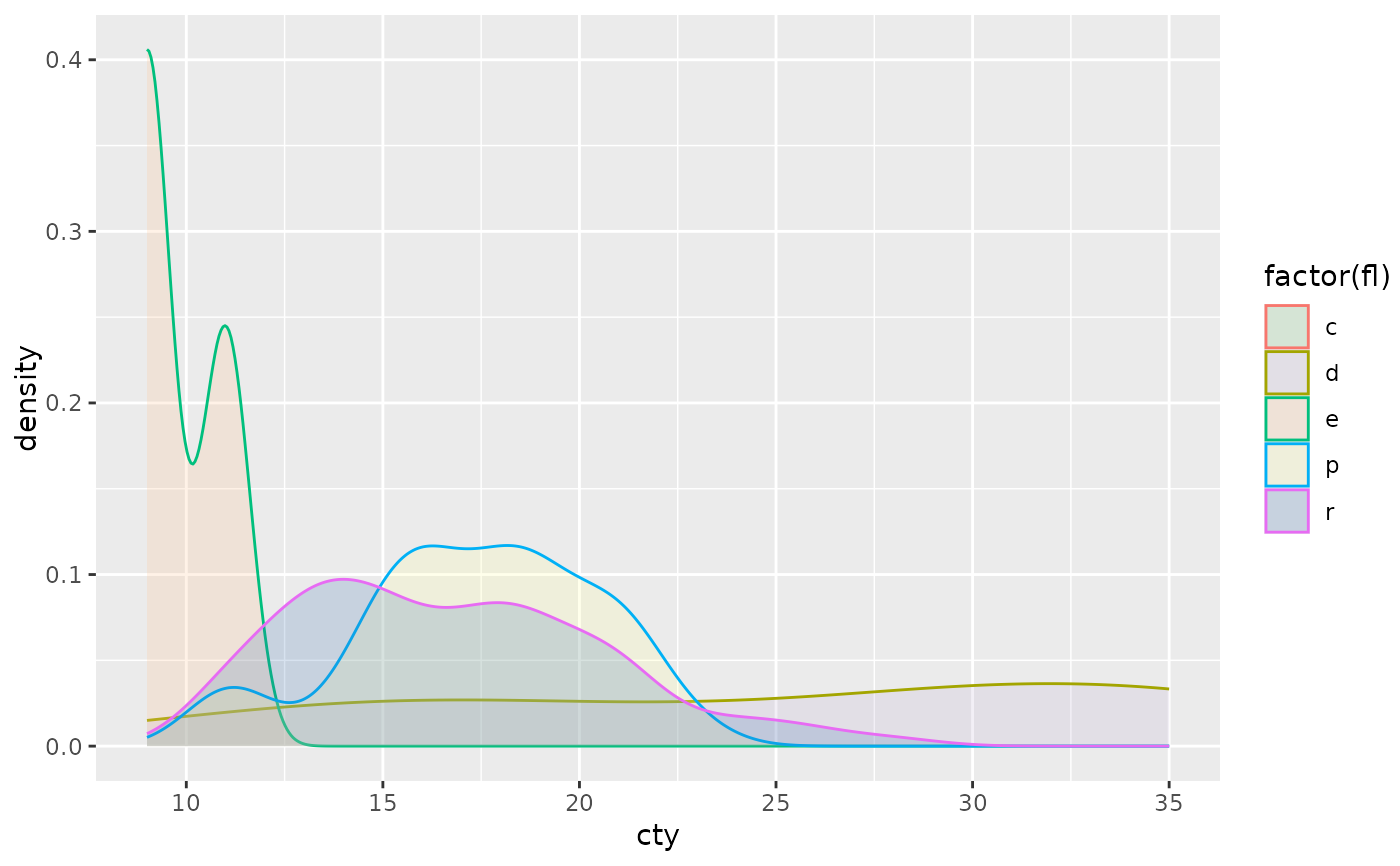The default discrete colour scale. Defaults to scale_fill_hue()/scale_fill_brewer() unless type (which defaults to the ggplot2.discrete.fill/ggplot2.discrete.colour options) is specified.

## Usage

scale_colour_discrete(..., type = getOption("ggplot2.discrete.colour"))

scale_fill_discrete(..., type = getOption("ggplot2.discrete.fill"))

## Arguments

...

Additional parameters passed on to the scale type,

type

One of the following:

• A character vector of color codes. The codes are used for a 'manual' color scale as long as the number of codes exceeds the number of data levels (if there are more levels than codes, scale_colour_hue()/scale_fill_hue() are used to construct the default scale). If this is a named vector, then the color values will be matched to levels based on the names of the vectors. Data values that don't match will be set as na.value.

• A list of character vectors of color codes. The minimum length vector that exceeds the number of data levels is chosen for the color scaling. This is useful if you want to change the color palette based on the number of levels.

• A function that returns a discrete colour/fill scale (e.g., scale_fill_hue(), scale_fill_brewer(), etc).

## Examples

# Template function for creating densities grouped by a variable
cty_by_var <- function(var) {
ggplot(mpg, aes(cty, colour = factor({{var}}), fill = factor({{var}}))) +
geom_density(alpha = 0.2)
}

# The default, scale_fill_hue(), is not colour-blind safe
cty_by_var(class)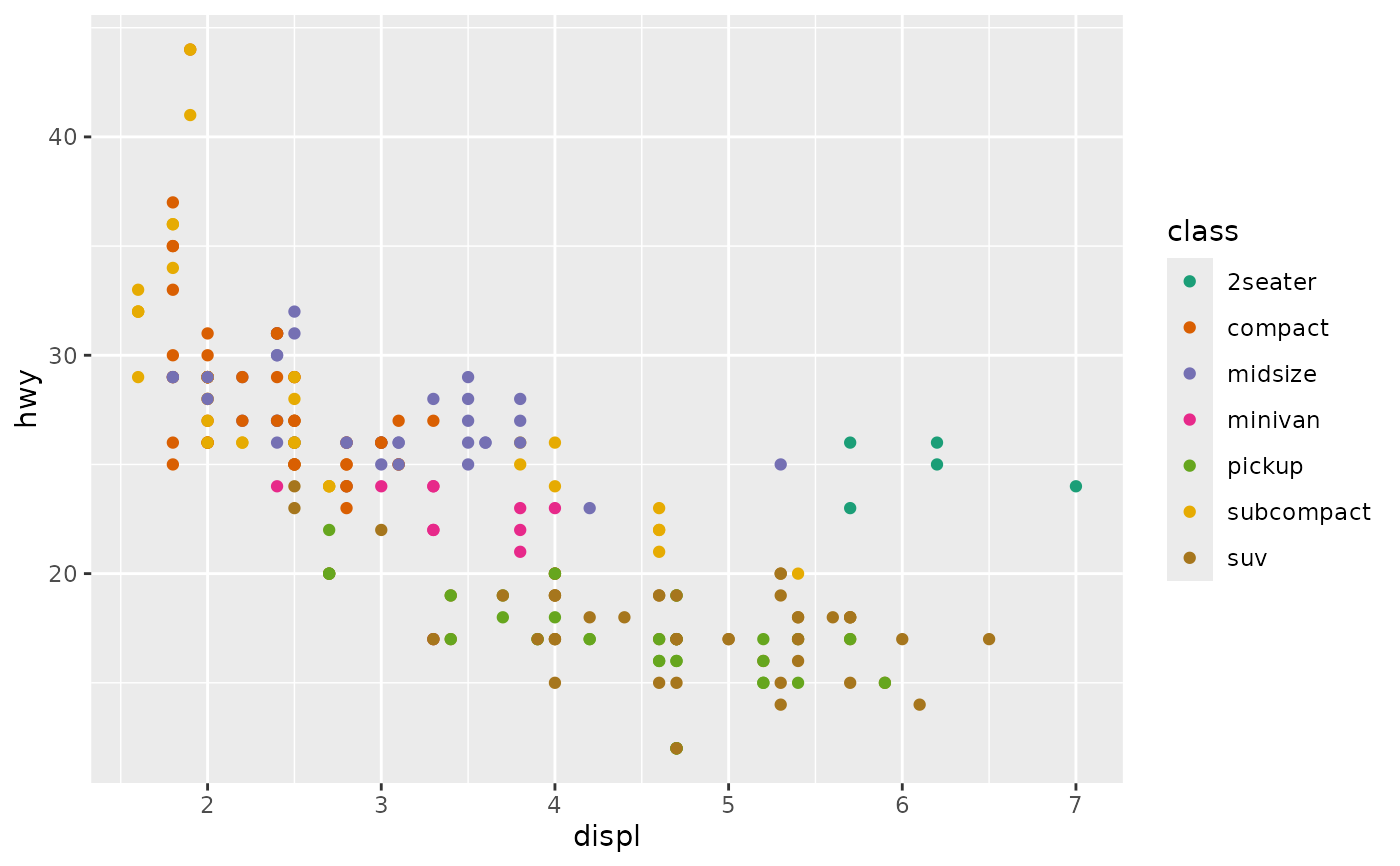# (Temporarily) set the default to Okabe-Ito (which is colour-blind safe)
okabe <- c("#E69F00", "#56B4E9", "#009E73", "#F0E442", "#0072B2", "#D55E00", "#CC79A7")
withr::with_options(
list(ggplot2.discrete.fill = okabe),
print(cty_by_var(class))
)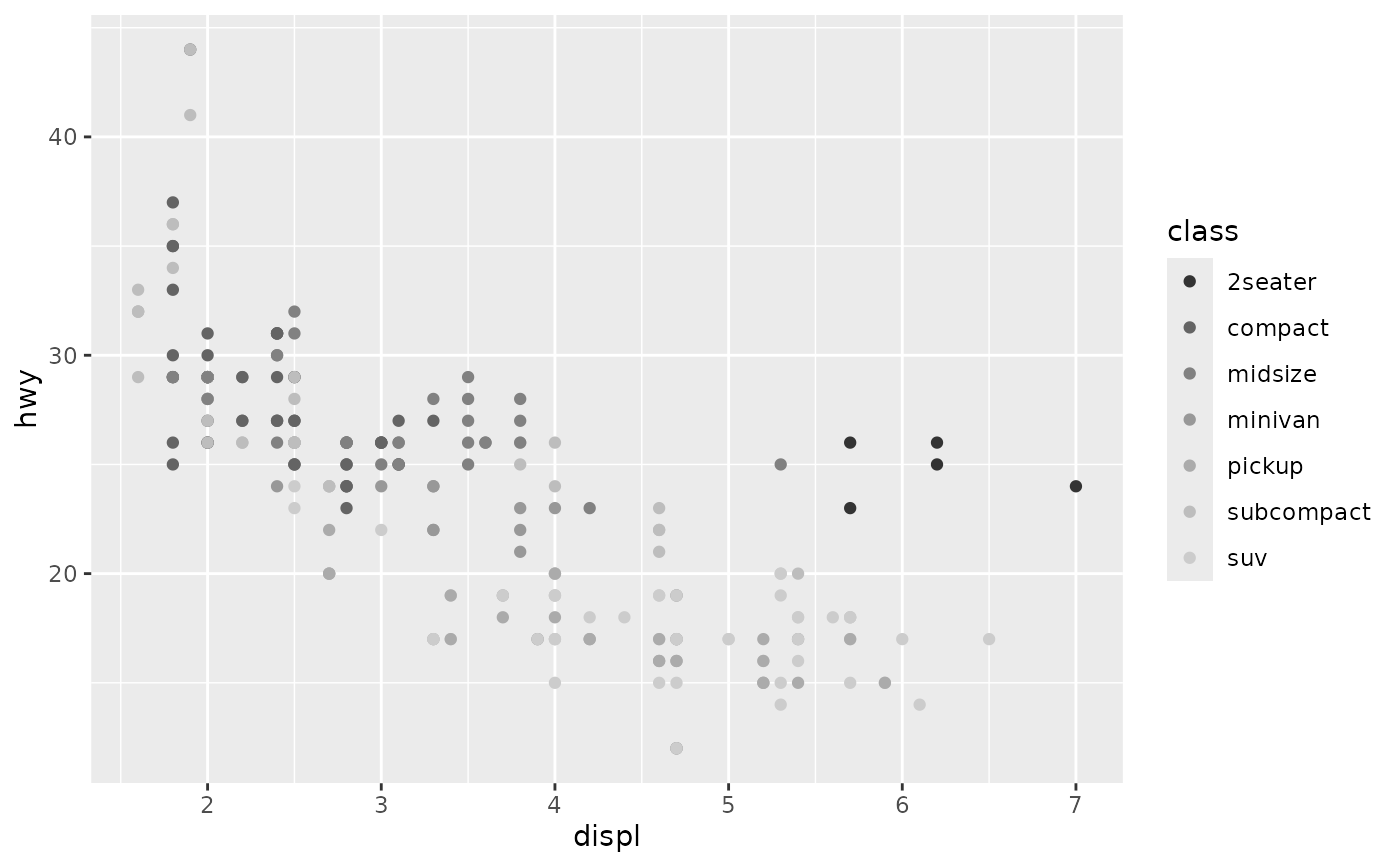# Define a collection of palettes to alter the default based on number of levels to encode
discrete_palettes <- list(
c("skyblue", "orange"),
RColorBrewer::brewer.pal(3, "Set2"),
RColorBrewer::brewer.pal(6, "Accent")
)
withr::with_options(
list(ggplot2.discrete.fill = discrete_palettes), {
# 1st palette is used when there 1-2 levels (e.g., year)
print(cty_by_var(year))
# 2nd palette is used when there are 3 levels
print(cty_by_var(drv))
# 3rd palette is used when there are 4-6 levels
print(cty_by_var(fl))
})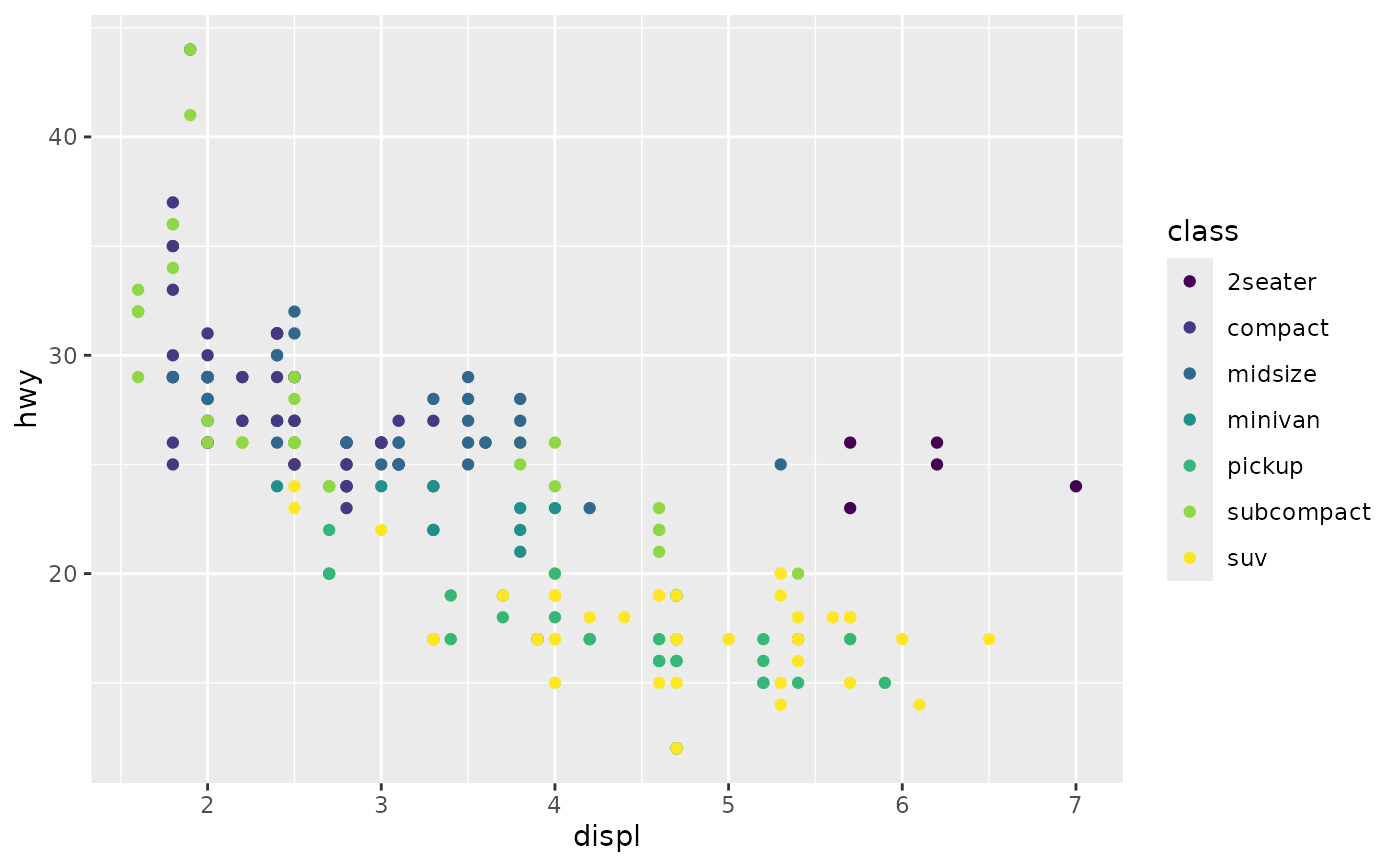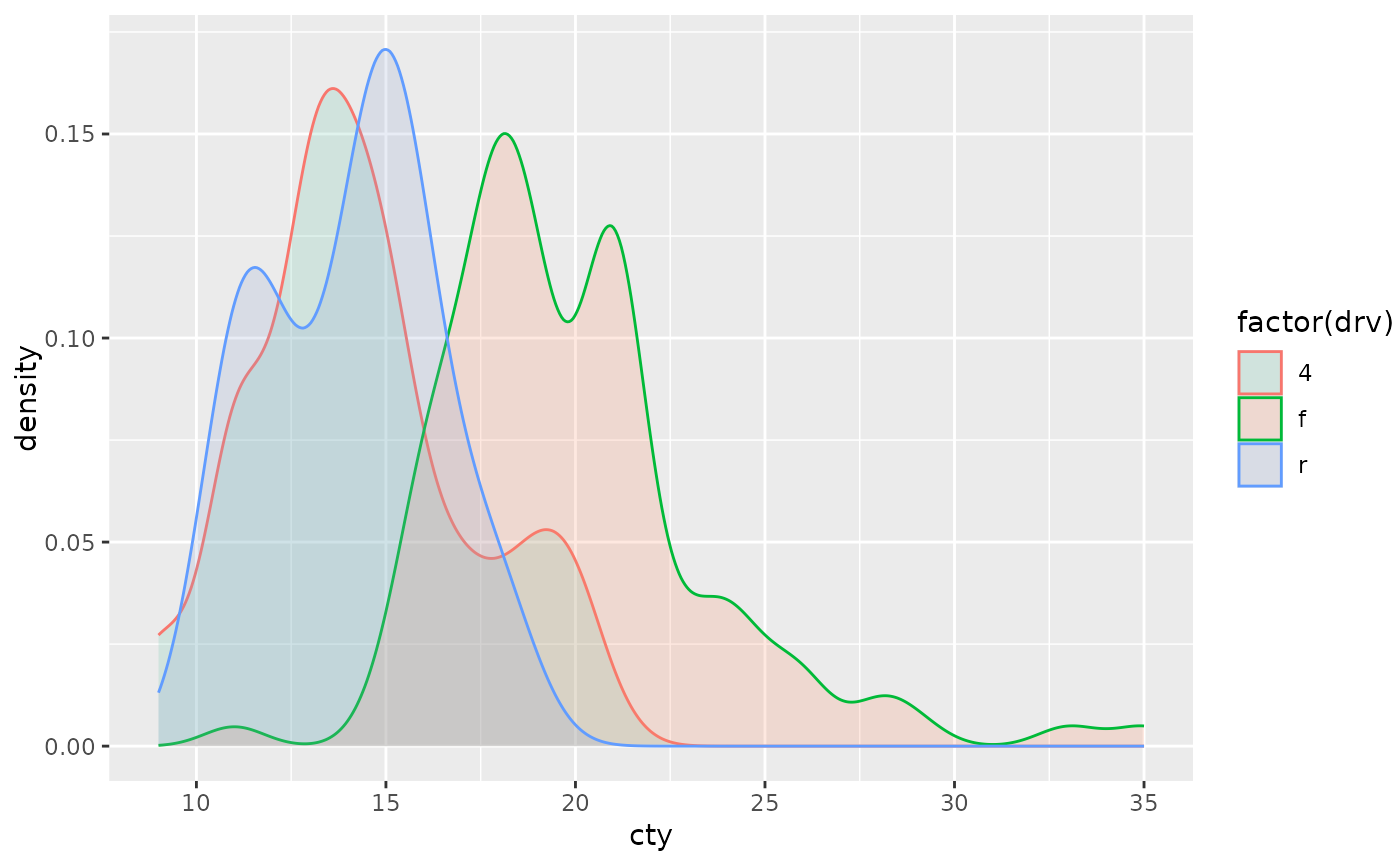#> Warning: Groups with fewer than two data points have been dropped.
#> Warning: no non-missing arguments to max; returning -Inf